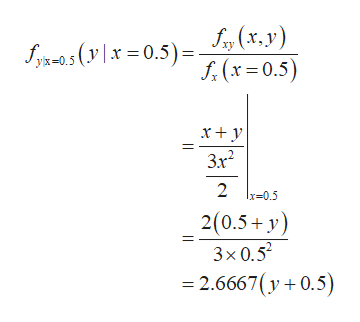# A joint probability density function is given by f(x,y) = x+y, 0<=x,y<=1. Find conditional expectation of y, given x = 0.5.

Question
2 views

A joint probability density function is given by f(x,y) = x+y, 0<=x,y<=1. Find conditional expectation of y, given x = 0.5.

check_circle

Step 1

A joint probability density function is given by,

Step 2

The marginal density function of x is calculated as follows:

Step 3

The conditional probability density f...help_outlineImage TranscriptioncloseS, (x,v) 5. (x = 0.5) Syx-s( \ x = 0.5) = Xy x+ y 3.x? 2 Ix=0.5 2(0.5+ y) 3x 0.5 = 2.6667(y+0.5) fullscreen

### Want to see the full answer?

See Solution

#### Want to see this answer and more?

Solutions are written by subject experts who are available 24/7. Questions are typically answered within 1 hour.*

See Solution
*Response times may vary by subject and question.
Tagged in
MathProbability

### Other